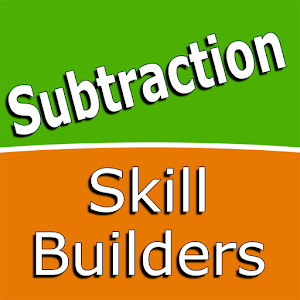\$2.49

# Android Giveaway of the Day - Subtraction Skill Builders

A math-based educational game.
\$2.49 期限切れ
ユーザーの評価: 0 (0%) 0 (0%)

このgiveawayオファーはすでに期限が切れています。Subtraction Skill Buildersが今紹介されています。

Intuitive interface powered by handwriting input and a fun and engaging mini-game in addition to a regular math trainer mode make our app stand out from the crowd of generic math learning apps.

With Subtraction Skill Builders you can practice and improve the following math skills:
- Subtracting 1
- Subtracting 2
- Subtracting 3
- Subtracting 4
- Subtracting 5
- Subtracting 6
- Subtracting 7
- Subtracting 8
- Subtracting 9
- Subtracting 10

Choose between two different modes:
- Math Trainer
- Math Shot mini-game

Math Trainer mode helps you to master the math facts, you can solve math problems at you own pace without any time constrain.

Math Shot is a math mini-game with fun and engaging game-play and as we all know learning through play and fun is more effective. The game difficulty adapts to player's skills and makes the game suitable for all ages. Math Shot helps students to develop math fact fluency - the result of consistent learning, repetition, and practice.

Sergey Malugin

Education

5.0.0

15M

Everyone

4.0 and up

## コメント Subtraction Skill Builders

Thank you for voting!